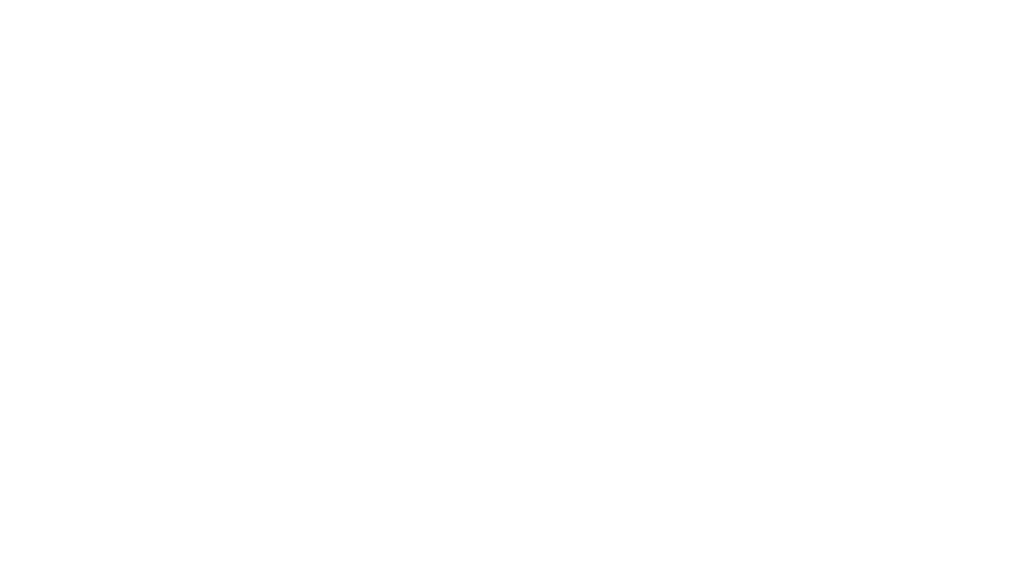# 13.3 Class 10 Maths NCERT Solutions PDF Download CBSE Exercise Book 2022

## 13.3 Class 10 Maths NCERT Solutions PDF Download Full Exercise Book 2022

13.3 Class 10 Maths NCERT Solutions PDF Download Full Exercise Book 2022 for CBSE and State Board Exam.

• 13.3 Class 10
• Exercise 13.3 Class 10
• Exercise 13.3 Class 10 Maths Solutions
• NCERT Solutions 13.3 Class 10
• Class 10 Exercise 13.3 Maths NCERT Solutions

## 13.3 Class 10 Maths NCERT Solutions

You can read full ebook pdf of class 10 for maths exercise 13.3. NCERT solutions are available with pdf book and answers are available free for all boards.

## Exercise: 13.1 (Page No: 244)

1. 2 cubes each of volume 64 cm3 are joined end to end. Find the surface area of the resulting cuboid.

The diagram is given as: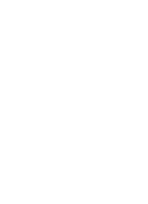Given,

The Volume (V) of each cube is = 64 cm3

This implies that a3 = 64 cm3

∴ a = 4 cm

Now, the side of the cube = a = 4 cm

Also, the length and breadth of the resulting cuboid will be 4 cm each. While its height will be 8 cm.

So, the surface area of the cuboid = 2(lb+bh+lh)

= 2(8×4+4×4+4×8) cm2

= 2(32+16+32) cm2

= (2×80) cm2 = 160 cm2

2. A vessel is in the form of a hollow hemisphere mounted by a hollow cylinder. The diameter of the hemisphere is 14 cm and the total height of the vessel is 13 cm. Find the inner surface area of the vessel.

The diagram is as follows: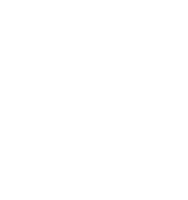Now, the given parameters are:

The diameter of the hemisphere = D = 14 cm

The radius of the hemisphere = r = 7 cm

Also, the height of the cylinder = h = (13-7) = 6 cm

And, the radius of the hollow hemisphere = 7 cm

Now, the inner surface area of the vessel = CSA of the cylindrical part + CSA of hemispherical part

(2πrh+2πr2) cm2 = 2πr(h+r) cm2

2×(22/7)×7(6+7) cm2 = 572 cm2

3. A toy is in the form of a cone of radius 3.5 cm mounted on a hemisphere of same radius. The total height of the toy is 15.5 cm. Find the total surface area of the toy.

The diagram is as follows: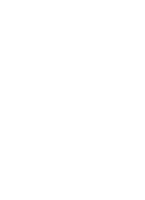Given that the radius of the cone and the hemisphere (r) = 3.5 cm or 7/2 cm

The total height of the toy is given as 15.5 cm.

So, the height of the cone (h) = 15.5-3.5 = 12 cm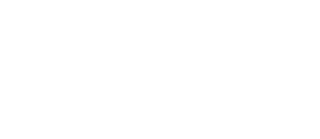∴ The curved surface area of cone = πrl

(22/7)×(7/2)×(25/2) = 275/2 cm2

Also, the curved surface area of the hemisphere = 2πr2

2×(22/7)×(7/2)2

= 77 cm2

Now, the Total surface area of the toy = CSA of cone + CSA of hemisphere

= (275/2)+77 cm2

= (275+154)/2 cm2

= 429/2 cm2 = 214.5cm2

So, the total surface area (TSA) of the toy is 214.5cm2

4. A cubical block of side 7 cm is surmounted by a hemisphere. What is the greatest diameter the hemisphere can have? Find the surface area of the solid.

It is given that each side of cube is 7 cm. So, the radius will be 7/2 cm.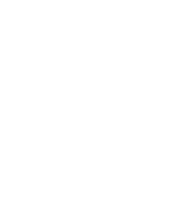We know,

The total surface area of solid (TSA) = surface area of cubical block + CSA of hemisphere – Area of base of hemisphere

∴ TSA of solid = 6×(side)2+2πr2-πr2

= 6×(side)2+πr2

= 6×(7)2+(22/7)×(7/2)×(7/2)

= (6×49)+(77/2)

= 294+38.5 = 332.5 cm2

So, the surface area of the solid is 332.5 cm2

5. A hemispherical depression is cut out from one face of a cubical wooden block such that the diameter l of the hemisphere is equal to the edge of the cube. Determine the surface area of the remaining solid.

The diagram is as follows: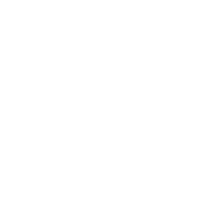Now, the diameter of hemisphere = Edge of the cube = l

So, the radius of hemisphere = l/2

∴ The total surface area of solid = surface area of cube + CSA of hemisphere – Area of base of hemisphere

TSA of remaining solid = 6 (edge)2+2πr2-πr2

= 6l2 + πr2

= 6l2+π(l/2)2

= 6l2+πl2/4

= l2/4(24+π) sq. units

6. A medicine capsule is in the shape of a cylinder with two hemispheres stuck to each of its ends. The length of the entire capsule is 14 mm and the diameter of the capsule is 5 mm. Find its surface area.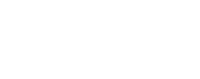Two hemisphere and one cylinder are shown in the figure given below.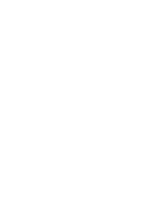Here, the diameter of the capsule = 5 mm

∴ Radius = 5/2 = 2.5 mm

Now, the length of the capsule = 14 mm

So, the length of the cylinder = 14-(2.5+2.5) = 9 mm

∴ The surface area of a hemisphere = 2πr2 = 2×(22/7)×2.5×2.5

= 275/7 mm2

Now, the surface area of the cylinder = 2πrh

= 2×(22/7)×2.5×9

(22/7)×45 = 990/7 mm2

Thus, the required surface area of medicine capsule will be

= 2×surface area of hemisphere + surface area of the cylinder

= (2×275/7) × 990/7

= (550/7) + (990/7) = 1540/7 = 220 mm2

7. A tent is in the shape of a cylinder surmounted by a conical top. If the height and diameter of the cylindrical part are 2.1 m and 4 m respectively, and the slant height of the top is 2.8 m, find the area of the canvas used for making the tent. Also, find the cost of the canvas of the tent at the rate of Rs 500 per m2. (Note that the base of the tent will not be covered with canvas.)

It is known that a tent is a combination of cylinder and a cone.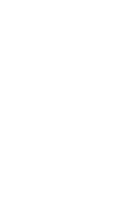From the question we know that

Diameter = 4 m

Slant height of the cone (l) = 2.8 m

Radius of the cone (r) = Radius of cylinder = 4/2 = 2 m

Height of the cylinder (h) = 2.1 m

So, the required surface area of tent = surface area of cone + surface area of cylinder

= πrl+2πrh

= πr(l+2h)

= (22/7)×2(2.8+2×2.1)

= (44/7)(2.8+4.2)

= (44/7)×7 = 44 m2

∴ The cost of the canvas of the tent at the rate of ₹500 per mwill be

= Surface area × cost per m2

44×500 = ₹22000

So, Rs. 22000 will be the total cost of the canvas.

8. From a solid cylinder whose height is 2.4 cm and diameter 1.4 cm, a conical cavity of the

same height and same diameter is hollowed out. Find the total surface area of the

remaining solid to the nearest cm2.

The diagram for the question is as follows: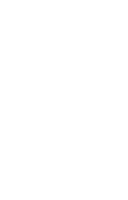From the question we know the following:

The diameter of the cylinder = diameter of conical cavity = 1.4 cm

So, the radius of the cylinder = radius of the conical cavity = 1.4/2 = 0.7

Also, the height of the cylinder = height of the conical cavity = 2.4 cm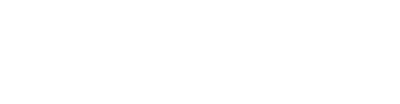Now, the TSA of remaining solid = surface area of conical cavity + TSA of the cylinder

= πrl+(2πrh+πr2)

= πr(l+2h+r)

= (22/7)× 0.7(2.5+4.8+0.7)

= 2.2×8 = 17.6 cm2

So, the total surface area of the remaining solid is 17.6 cm2

13.3 Class 10 Maths, 13.3 Class 10 Maths, 13.3 Class 10 Maths, 13.3 Class 10 Maths pdf download

## 13.3 Class 10 Maths, 13.3 Class 10 Maths pdf, 13.3 Class 10 Maths ncert solutions, 13.3 Class 10 Maths solutions, Ex 13.3 Class 10 Maths.

13.3 Class 10 Maths, 13.3 Class 10 Maths pdf, 13.3 Class 10 Maths ncert solutions, 13.3 Class 10 Maths solutions, Ex 13.3 Class 10 Maths pdf download for all CBSE and State board exam 2022.